Our prices are denominated in USD.

We ship internationally!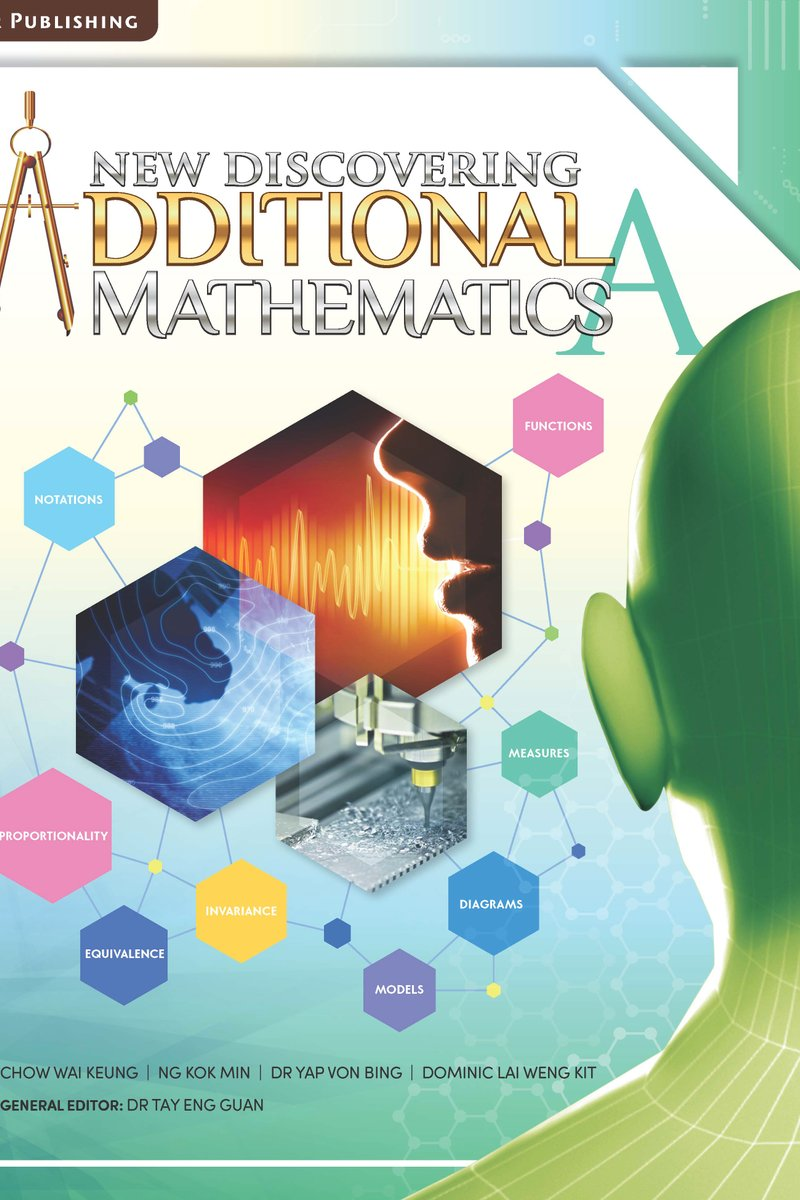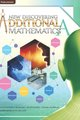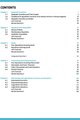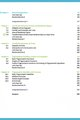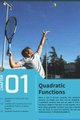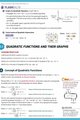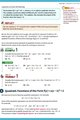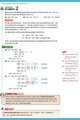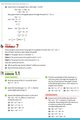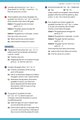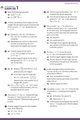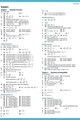# New Discovering Additional Mathematics Textbook A

US\$17.35

New Discovering Additional Mathematics is a set of textbooks written with learner-centric approach that builds upon the knowledge and skills learnt in elementary mathematics. It is based on the latest O-Level and N(A)-Level Additional Mathematics Syllabus implemented from 2020 by Ministry of Education of Singapore.

Illustrative examples and diagrams help students understand and apply the mathematical concepts that have been learnt. The examples are also carefully selected to promote discussions on relevant Big Ideas in mathematics and modelling of essential problem-solving strategies.

The contents of the syllabus are organised under three strands:

1. Algebra
2. Geometry & Trigonometry
3. Calculus

Key Features

1. Learning Objectives
Provided at the start of each section to inform students about what they will be learning in the section.

2. Connecting Ideas
Emphasise the links between different mathematical concepts and the Big Ideas of mathematics, mathematics and other disciplines as well as between mathematics and the real world.

3. Worked Examples
Facilitates students' understanding of concepts and shows how ideas and reasoning can be expressed clearly in mathematical language.

4. Try it Yourself
Enables students to practise what have been just learnt in Worked Examples.

5. Practice Exercise
• Basic Mastery : Simple and direct application of a particular concept or skills
• Intermediate: Application of a few concepts and/or combination of skills
• Advanced: Higher-order thinking and the application of several concepts or skills, covered within the chapter or across different topics.

6. Let's Sum Up
Consolidates important concepts in the chapter

7. Flashback + Reflect + Extend Your Learning Curve

8. Core Vocabulary
Highlights key terms from the chapter

9. Getting Started
Provides relevant real-life applications of concepts in the immediate chapter and acts as a trigger that piques students' interest

10. Integrated Exercise
Requires students to synthesise concepts learnt across different chapters.

11. Spotlight
Draws attention to important mathematical information.

12. Coding
Provides coding opportunities to support development of computational thinking.

13. Extra Info
Provides extra information such as scientific, historical and fun facts to pique students' interest.

Graphs, Always Negative or Positive, Models

2. Equations and Inequalities
Nature of roots, Simultaneous Equations, Quadratic Inequalities

3. Surds
Four Operations and Equations

INTEGRATED EXERCISE

4. Polynomials and Partial Fractions
Remainder and Factor Theorems, Cubic Polynomials and Equations, Partial Fractions

5. Exponential and Logarithmic Functions and Equations

6. Binomial Expansions

7. Coordinate Geometry of Lines and Rectilinear Figures
Midpoint, Parallel and Perpendicular Lines, Area

8. Coordinate Geometry of Circles
Equation, Intersection Points

9. Trigonometric Functions, Graphs and Equations

10. Trigonometric Identities and Formulae
Basic Identities, Addition, Double Angle , R Formulae

INTEGRATED EXERCISE